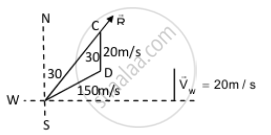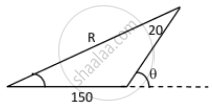Department of Pre-University Education, KarnatakaPUC Karnataka Science Class 11
Advertisement Remove all ads

# An Aeroplane Has to Go from a Point a to Another Point B, 500 Km Away Due 30° East of North. a Wind is Blowing Due North at a Speed of 20 M/S. Find the Time Taken by the Plane to Go from a to B. - Physics

Sum

An aeroplane has to go from a point A to another point B, 500 km away due 30° east of north. A wind is blowing due north at a speed of 20 m/s. The air-speed of the plane is 150 m/s. Find the time taken by the plane to go from A to B.

Advertisement Remove all ads

#### Solution

Given:
Distance between points A and B = 500 km
B from A is 30˚ east of north.
Speed of wind due north, vw = 20 m/s
Airspeed of the plane, va = 150 m/s
Let $\vec{R}$  be the resultant direction of the plane to reach point B.Time taken by the plane to reach point B from point A: $\sin^{- 1} \left( \frac{1}{15} \right) = 3^\circ 48'$

⇒ 30° + 3°48' = 33°48

$R = \sqrt{A^2 + B^2 + 2AB\cos\theta}$

$R = \sqrt{150 + 20 + 2\left( 150 \right)\left( 20 \right) \cos\left( 33^\circ48' \right)}$

$= \sqrt{27886} = 167 \text{ m/s }$

$\text{ Time } = \frac{s}{v} = \frac{500000}{167}$

$= 2994 s = 49 . 0 \approx 50 \text{ minutes }$

Is there an error in this question or solution?
Advertisement Remove all ads

#### APPEARS IN

HC Verma Class 11, 12 Concepts of Physics 1
Chapter 3 Rest and Motion: Kinematics
Q 49.2 | Page 54
Advertisement Remove all ads
Advertisement Remove all ads
Share
Notifications

View all notifications

Forgot password?